# Direct photon elliptic flow in Pb-Pb collisions at $\sqrt{s_{\rm NN}}$ = 2.76 TeV

The elliptic flow of inclusive and direct photons was measured at mid-rapidity in two centrality classes 0-20% and 20-40% in Pb-Pb collisions at $\sqrt{s_{\rm NN}}$ = 2.76 TeV by ALICE. Photons were detected with the highly segmented electromagnetic calorimeter PHOS and via conversions in the detector material with the $e^{+}e^{-}$ pairs reconstructed in the central tracking system. The results of the two methods were combined and the direct photon elliptic flow was extracted in the transverse momentum range $0.9 <~ p_{\rm T} <~ 6.2$ GeV/$c$. A comparison to RHIC data shows a similar magnitude of the measured direct-photon elliptic flow. Hydrodynamic and transport model calculations are systematically lower than the data, but are found to be compatible.

Figures

## Figure 1

 Comparison of the measured inclusive photon flow $(\vincg)$ to the individual PCM and PHOS measurements $(v_{2}^{\rm \gamma, ind})$ in the 0--20\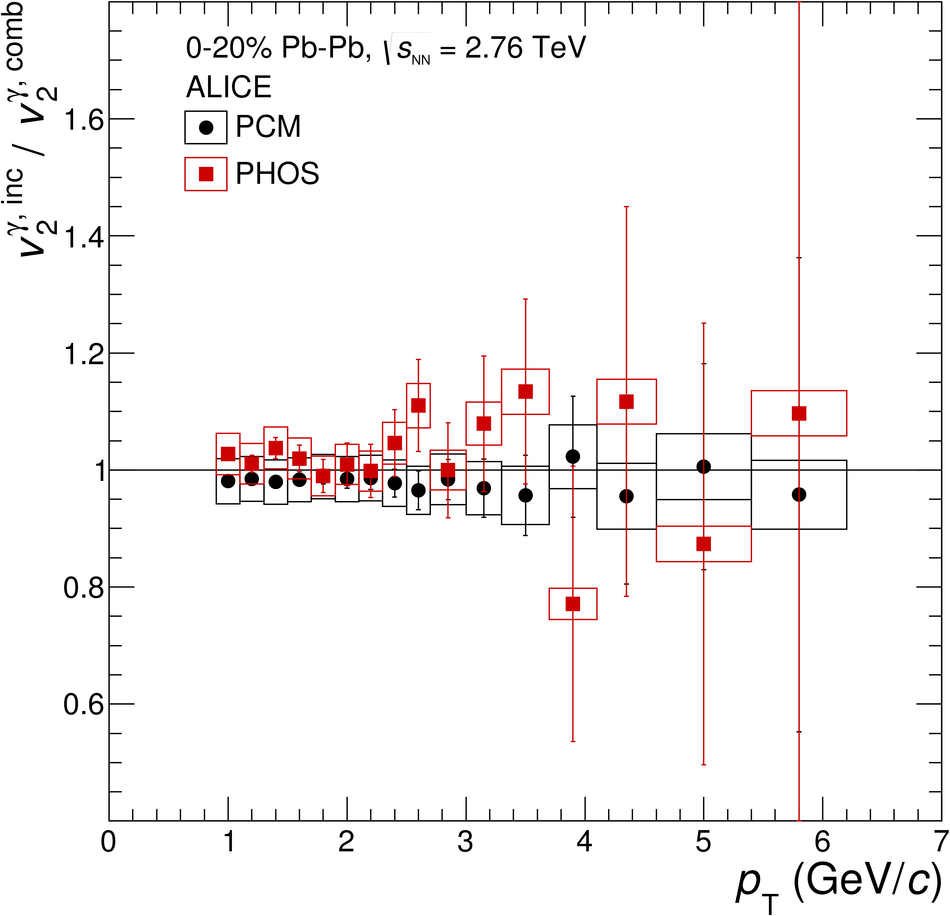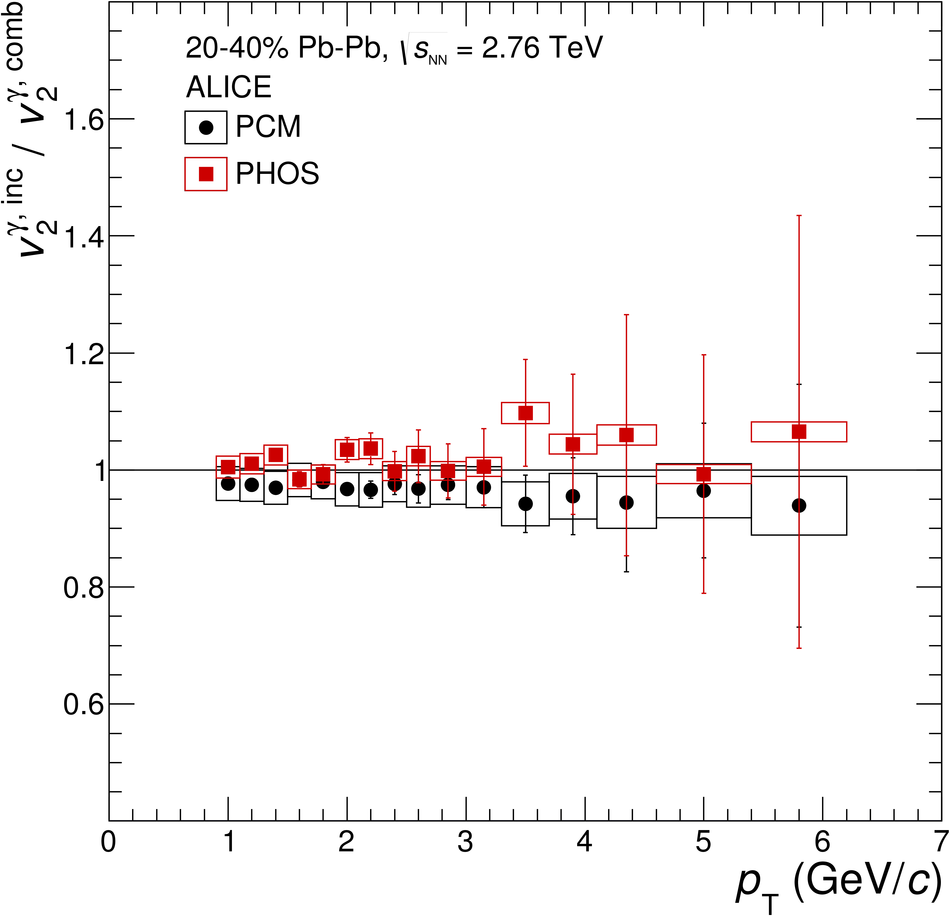## Figure 2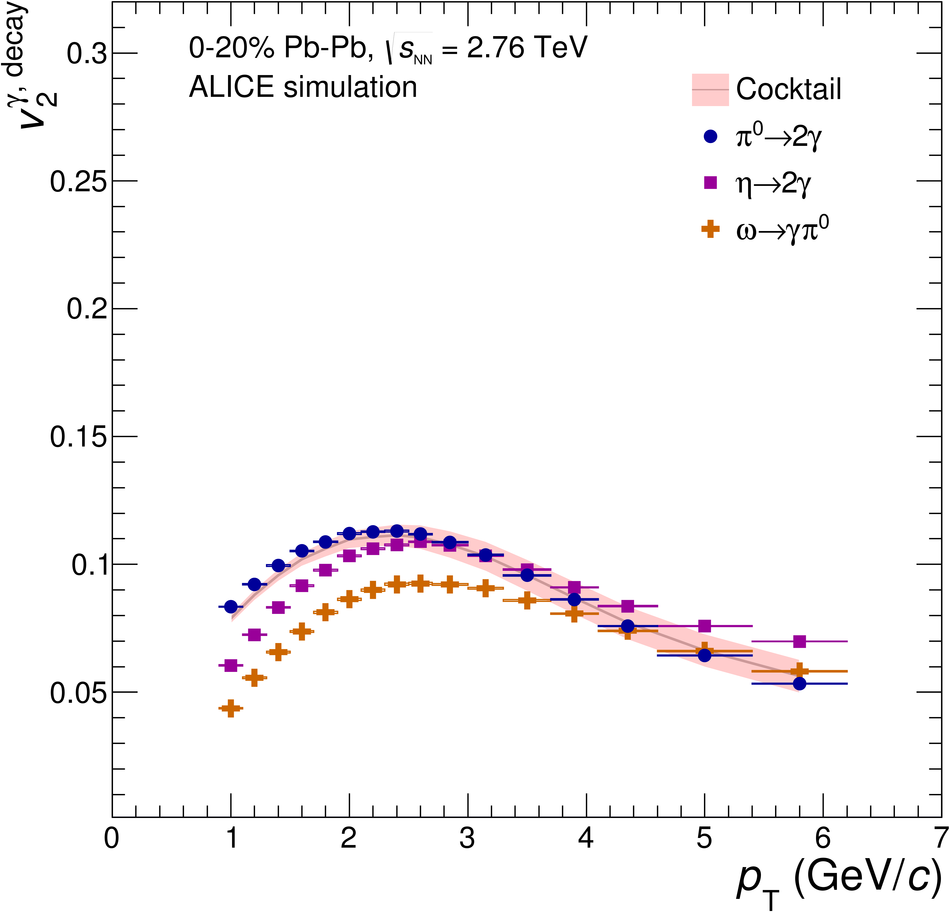## Figure 3

 Elliptic flow of inclusive photons and decay photons, compared to hydrodynamic and transport PHSD model predictions in the 0--20\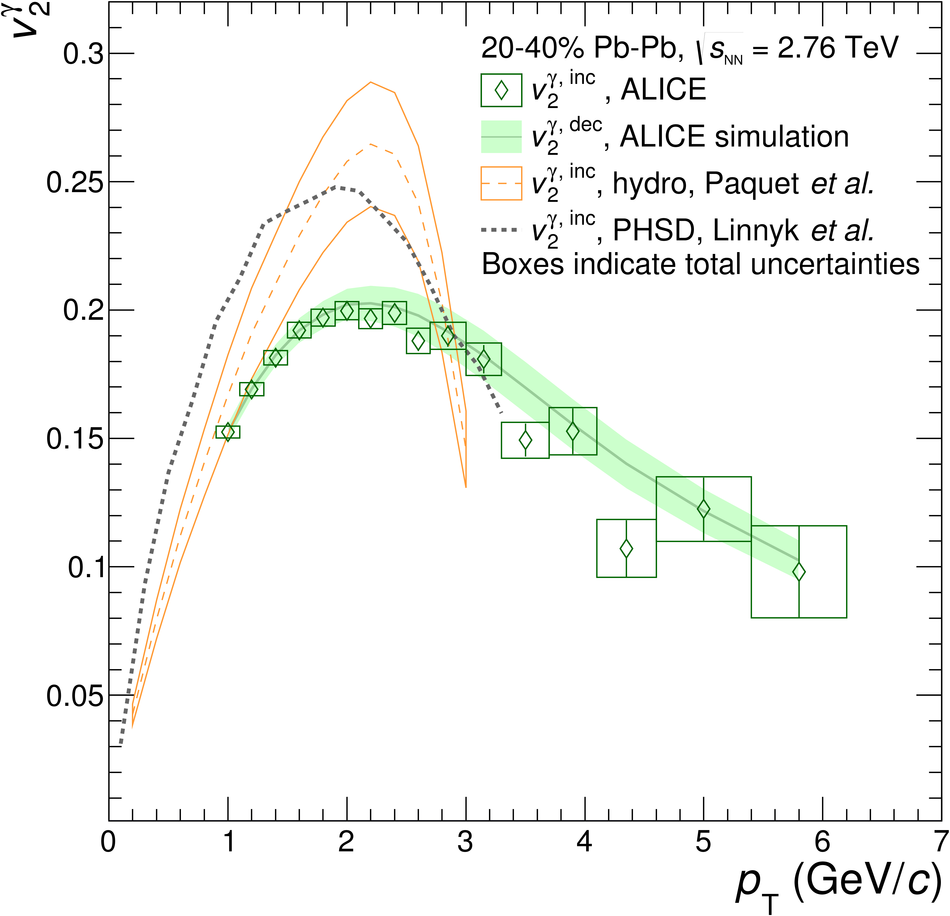## Figure 4

 Left: Central value (solid red line) and uncertainty of the direct-photon $v_2$ for a selected $\pT$ interval. The upper and lower edges of the red shaded area correspond to the total uncertainty of $\vdirg$ as obtained from linear Gaussian propagation of the uncertainties $\sigma(\vincg)$ and $\sigma(\vdecg)$. The Gaussian (with arbitrary normalization) reflects the measured value of $\Rg$ in this $\pT$ interval (blue dashed line) and its $\pm 1\sigma$ uncertainty (dark-blue shaded interval). Right: Posterior distribution of the true value of $\vdirg$ for the same interval in the Bayesian approach Note that the distribution has a non-Gaussian shape, implying that the $\pm$2$\sigma$ interval typically corresponds to a probability of less than 95.45\## Figure 5

 Elliptic flow of direct photons compared with PHENIX results for the 0--20\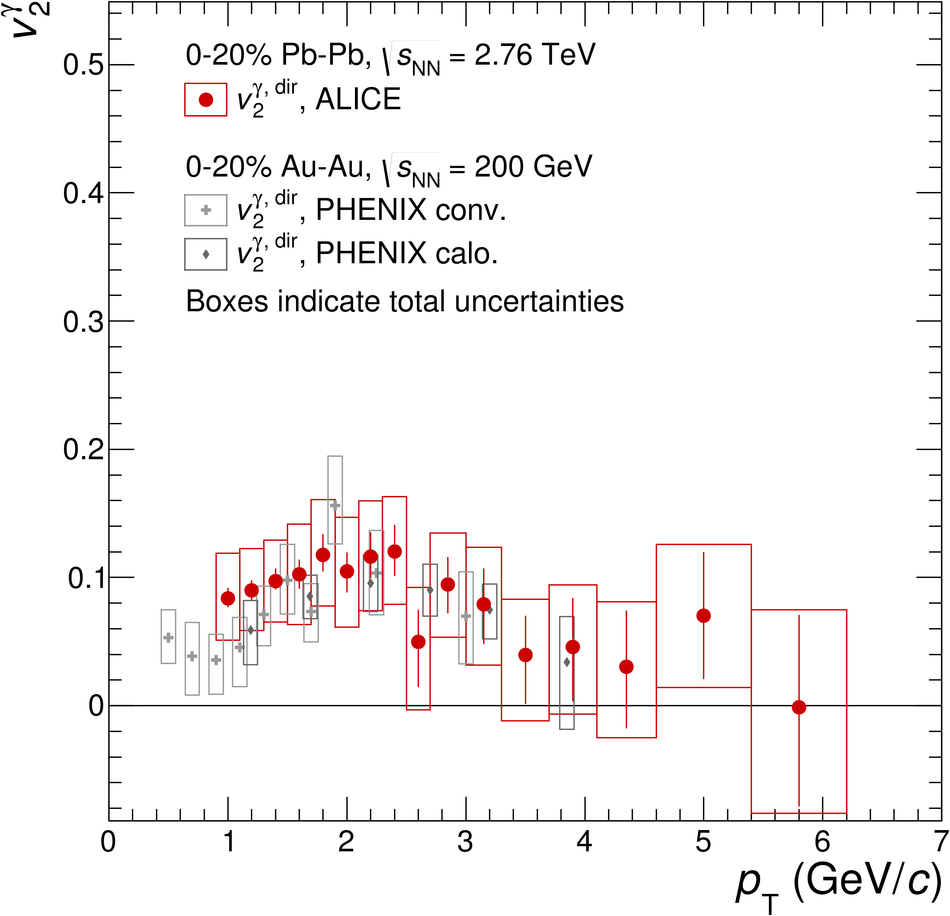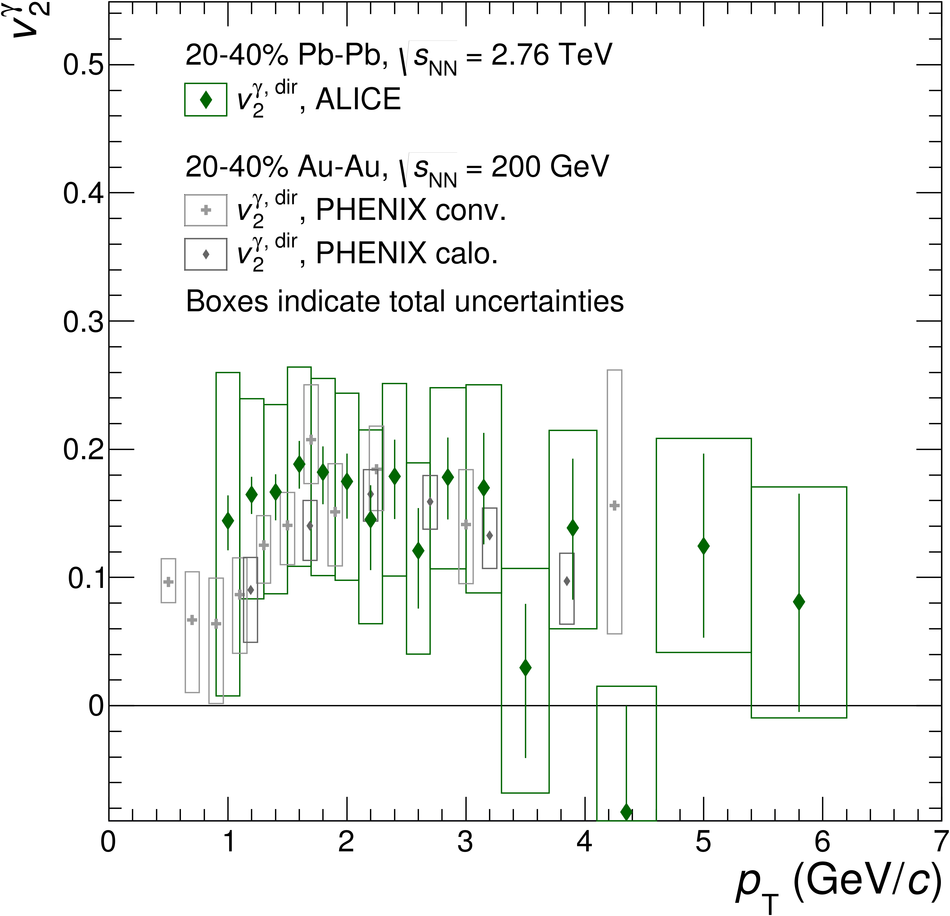## Figure 6

 Elliptic flow of direct photons compared to model calculations in the 0--20\